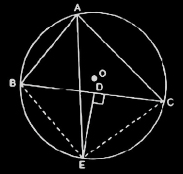Guru

# In any triangle ABC, if the angle bisector of ∠A and perpendicular bisector of BC intersect, prove that they intersect on the circumcircle of the triangle ABC. Q.10

• 0

I want to know the best answer of the question from Circles chapter of class 9th ncert math. The question from exercise 10.6of math. Give me the easy way for solving this question of 10 In any triangle ABC, if the angle bisector of ∠A and perpendicular bisector of BC intersect, prove that they intersect on the circumcircle of the triangle ABC.

Share

1. Consider this diagramHere, join BE and CE.

Now, since AE is the bisector of ∠BAC,

∠BAE = ∠CAE

Also,

∴arc BE = arc EC

This implies, chord BE = chord EC

Now, consider triangles ΔBDE and ΔCDE,

DE = DE     (It is the common side)

BD = CD     (It is given in the question)

BE = CE      (Already proved)

So, by SSS congruency, ΔBDE ΔCDE.

Thus, ∴∠BDE = ∠CDE

We know, ∠BDE = ∠CDE = 180°

Or, ∠BDE = ∠CDE = 90°

∴ DE ⊥ BC (hence proved).

• 0